• C++指针

千次阅读 多人点赞 2021-05-03 10:51:12
C++ 指针介绍 (对象指针 & this 指针)

概述

指针 (pointer) 是一个变量, 其指为另一个变量的地址. 即内存位置的直接地址.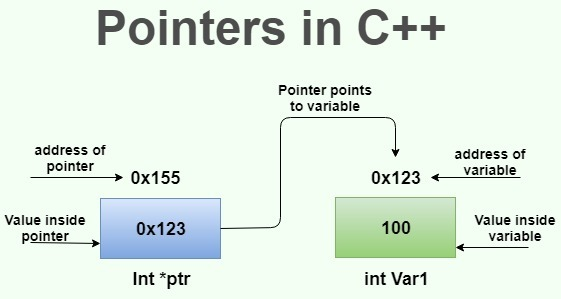指向对象的指针

在建立对象时, 编译系统会为每一个对象分配一定的存储空间, 以存放其成员.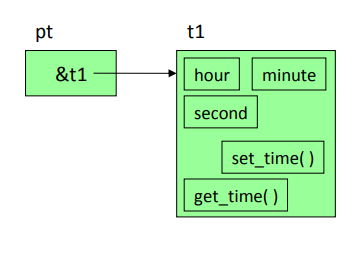我们可以定义一个指针变量, 用来存放对象的指针. 例如:

Time time1;
Time *p;  // 定义指针, 格式: 类名 *对象指针名
p = &time1;  // 将指针指向Time类对象

我们可以通过对象指针访问对象和对象的成员. 例如:

int main() {

Time time1;
Time *p;  // 定义指针, 格式: 类名 *对象指针名
p = &time1;  // 将指针指向Time类对象

p->hour; // 等同于time1.hour
p->show_time();  // 等同于time1.show_time()

return 0;
}

指向对象数据成员的指针

对象中的成员也有地址, 存放对象成员地址的指针变量就是指向对象成员的指针变量.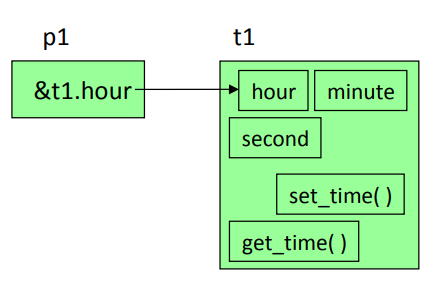定义指向对象数据成员的指针变量的方法和定义指向不同变量的指针变量方法相同. 例如:

int main() {

Time time1;
int *p;  // 定义指针, 格式: 类名 *对象指针名

p = &time1.hour;  // 将指针指向time1对象的hour成员

return 0;
}

通过指向对象数据成员的指针变量访问成员. 例如:

int main() {

Time time1;
int *p;  // 定义指针, 格式: 类名 *对象指针名

p = &time1.hour;  // 将指针指向time1对象的hour成员
cout << *p << endl;  // *p等同于time1.hour

return 0;
}

this 指针

每个对象都可以利用一个自己的特殊指针 this, 即指向当前对象的指针.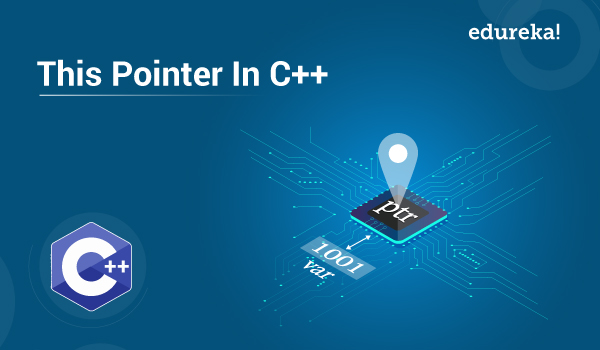Box 类:

#ifndef PROJECT1_BOX_H
#define PROJECT1_BOX_H

class Box {
private:
double height;
double width;
double length;
public:
Box();
Box(double h, double w, double l);
double volume();
};

#endif //PROJECT1_BOX_H

Box.cpp:

#include "Box.h"

Box::Box() : height(-1), width(-1), length(-1) {}

Box::Box(double h, double w, double l) : height(h), width(w), length(l) {}

double Box::volume(){
return (height * width * length);
}

mian:

#include "Box.h"
#include <iostream>
using namespace std;

int main() {

Box a(2,2,2);
double volume = a.volume();

cout << "Box 体积: " << volume << endl;

return 0;
}

this 指针的作用

调用 a.volume(), this 值为对象 a 起始地址, 实际执行:

return ((*this).height * (*this).width * (*this).length);
return (this -> height) * (this -> width) * (this - >length)
return (a.height) * (a.width) * (a.length)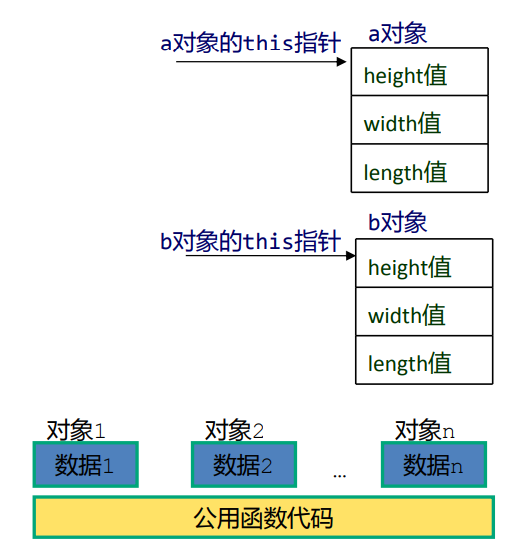this 指针的实现

C++ 在处理时, 会在成员函数的形参列中增加一个 this 指针. 调用时, 将对象的地址给形参 this 指针, 然后按 this 的指向去引用其他成员.

程序中的调用:
a.volume();

实际的调用方式是:
a.volume(&a);

展开全文• 不止C++才有指针，Golang也有指针呦！二者有哪些区别呢？今天就来看一看。 正文 什么是指针指针是用来指向任何一个变量的内存地址的类型，它所指向的变量的内存地址在 32 和 64 位机器上分别占用 4 或 8 ...

目录

前言

正文

什么是指针？

指针怎么用？

明显差异

结论

前言

不止C++有指针，Golang也有指针呦！二者有哪些区别呢？今天就来看一看。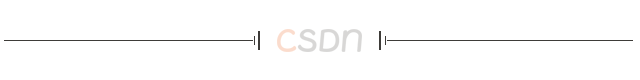正文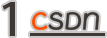什么是指针？

指针是用来指向任何一个变量的内存地址的类型变量，它所指向的变量的内存地址在 32 和 64 位机器上分别占用 4 或者 8 个字节，占用字节的大小与所指向的值的大小无关。

Golang

下面我们通过一个例子，来了解在Golang语言中是如何获取变量的地址的。

实例代码：

package main

import (
"fmt"
)

func main() {
var a int = 1 // 一个整型变量a
var b string = "abcdefg" // 一个字符串变量b
fmt.Printf("%p %p", &a, &b) // 分别输出a和b的地址
}

上述代码的运行结果：

0xc00007e020 0xc00005e1e0

值得注意的是，Golang中在声明变量时，是可以使用汉字的（这一点确实比C++要秀）。

比如，下面的编码形式也是可以编译通过并且正确运行的，习惯了C++开发的朋友要是看到，肯定强迫症都要犯了。。。“我的意大利炮呢？”

package main

import (
"fmt"
)

func main() {
var 一个整型变量 int = 1
var 一个字符串变量 string = "abcdefg"
fmt.Printf("%p %p", &一个整型变量, &一个字符串变量)
}

上述代码的运行结果：

0xc000016060 0xc000010210

C++

下面我们通过一个例子来了解C++语言中是如何获取变量的地址的。

实例代码：

#include <iostream>
using namespace std;

int main() {
int a  = 1; // 一个整型变量a
string b  = "abcdefg"; // 一个字符串变量b
cout<< &a <<" "<< &b <<endl; // 分别输出a和b的地址
return 0;
}

上述代码的运行结果：

0x7ffea42614bc 0x7ffea42614c0

说真的，对比上面的两部分代码，感觉C++和Golang还是差不太多的，可能更多的还是语法上的差异。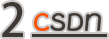指针怎么用？

指针可以用来获取变量的地址，同时也可以用来获取变量对应的值。正确使用指针无论是在Golang中还是C++中，都是一件非常有趣且高效的事情。想要成为一名优秀的Golang开发工程师或者C++开发工程师，学习好指针的使用都是非常有必要的。

Golang

下面我们通过一个例子，来了解Golang中的指针是如何获取变量地址以及它的值的。

实例代码：

package main

import (
"fmt"
)

func main() {

// 准备一个字符串类型
var a = "Hello, world"

// 获取字符串的地址, ptr类型为*string
ptr := &a

// 打印ptr的类型
fmt.Printf("ptr type: %T\n", ptr)

// 打印ptr的地址

// 对指针进行取值
value := *ptr

// 打印value的类型
fmt.Printf("value type: %T\n", value)

// 打印value的值
fmt.Printf("value: %s\n", value)

}

上述代码的运行结果：

ptr type: *string
value type: string
value: Hello, world

通过代码的执行结果，我们可以知道ptr是一个string字符串类型的指针，它的地址是0xc000010210，它指向的值是 Hello, world。

C++

#include <iostream>
using namespace std;

int main() {

// 准备一个字符串类型
string a = "Hello, world";

// 获取字符串的地址, ptr类型为*string
string* ptr = &a;

// 打印ptr的地址

// 对指针进行取值
string value = *ptr;

// 打印value的值
cout<<"value: " << value <<endl;

return 0;
}

上述代码的运行结果：

value: Hello, world

整体感觉，Golang和C++的差别不大。

明显差异

通过上边的对比分析，我们可以发现Golang和C++很多地方都很相似。那么，它们二者之间有没有明显的区别呢？

答案是有的！

C++语言中可以直接对指针做算术运算（+、-、++、--），但是Golang中是不行的。

接下来看两段代码实例：

Golang

package main

import (
"fmt"
)

func main() {

// 准备一个字符串类型
var a = "Hello, world"

// 获取字符串的地址, ptr类型为*string
ptr := &a

// 打印++ptr的地址

// 打印ptr+1的地址

}

上述代码的第16行和第19行都会报错，但是相同的操作在C++中都是可以的。

C++

#include <iostream>
using namespace std;

int main() {

// 准备一个字符串类型
string a = "Hello, world";

// 获取字符串的地址, ptr类型为*string
string* ptr = &a;

// 打印++ptr的地址

// 打印ptr+1的地址

return 0;
}

上述代码的运行结果：

通过对比结果，我们可以看出Golang中不支持对指针进行算术运算，而这一点在C++中没有限制。

结论

今天只是非常基础的介绍指针在Golang和C++中的使用方法，在实际开发中会涉及很多其他类型的指针变量，比如数组指针、指针数组、对象指针等。这篇文章算是抛砖引玉吧，获悉更多C++和Golang内容，欢迎评论关注！公众号“玩转音视频”，分享超实用的音视频技能！

展开全文指针 c++ go golang 编程语言
• C++指针操作

2021-01-14 12:55:15
C++指针操作 指针的特点：一、指针本身是一个对象，允许对指针赋值和拷贝，它可以先后指向几个不同的对象。二、指针无需在定义时赋初值，但会拥有一个不确定的值。因此，指针常常引发错误 1.引用 引用为对象起了另外...

C++指针操作

指针的特点：一、指针本身是一个对象，允许对指针赋值和拷贝，它可以先后指向几个不同的对象。二、指针无需在定义时赋初值，但会拥有一个不确定的值。因此，指针常常引发错误

1.引用

引用为对象起了另外一个名字，即下文refVal也是ival。

int ival = 1024int &refVal = ival;//refVal指向ival(是ival的另一个名字）
int &refVal2;//报错；引用必须被初始化

一旦初始化完成，refVal将和ival一直绑在一起，且refVal无法重新绑定在另外的对象上。

2.指针初始化

初始化，获取对象地址，利用指针访问对象

int ival = 42;
int *p = &ival;//p存放变量ival的地址，或者说p是指向变量ival的指针
cout<<*p<<endl;//由符号*得到指针p所指向的对象，输出42
*p=0;//由符号*得到指针p所指的对象，即可经由p为变量为ival赋值
cout<<*p<<endl;//输出0

p赋值实际上是为ival赋值
“&”取地址符，
“ * ” 解引用符

3.空指针

生成空指针的方法

int *p1 = nullptr;	//等价于int *p1 = 0;
int *p2 = 0;		//直接将p2初始化为字面常量0
int *p3 = NULL;		//等价于int *p3 = 0;需要先#include cstdlib

①c++11新标准，推荐使用。nullptr是一种特殊类型的字面值，它可以被转换成任意的其他指针类型
②NULL为预处理变量，在头文件cstdlib中定义，值为0

4.void*指针

void*是一种特殊的指针类型，用于存放任意对象的地址，但我们对该地址中到底是什么类型的对象并不了解。

double obj = 3.14,*pd = &obj;
void *pv = &obj;	//obj可以是任意类型的对象
pv = pd;			//pv可以存放任意类型的指针

void可以做的事：拿它和别的指针比较、作为函数的输入或输出、或者赋给另外一个void指针。
不能直接操作void*指针所指的对象

5.指向指针的指针

“ ** ” 表示指向指针的指针，“ *** ”表示指向指针的指针的指针

int ival = 1024;
int *pi = &ival;//指向int型的指针
int **ppi = &pi;//指向int型指针的指针
cout<<ival<<"\n"<<*pi<<"\n"<<**ppi<<endl;

输出全为ival的值6.指向指针的引用

引用本身不是一个对象，因此不能定义指向引用的指针。但指针是对象，所以存在对指针的引用

int i = 42;
int *p;			//p是一个int型指针
int *&r = p;	//r是一个对指针p的引用

r = &i;			//r引用了一个指针，因此给r赋值&i就是令p指向i
*r = 0;			//解引用r得到i,也就是p指向的对象，将i的值改为0

要理解r的类型到底是什么，最简单的办法是从右向左阅读r的定义。离变量名最近的符号（此例中是&r的符号&）对变量的类型有最直接的影响，因此r是一个引用。声明符的其余部分用以确定r引用的类型是什么，此例中的符号 * 说明r引用的是一个指针。最后，声明的基本数据类型部分指出r引用的是一个int指针。

面对一条比较复杂的指针或引用的声明语句时，从右向左阅读有助于弄清楚它的真实含义。

7.指针表达式

初始化

char ch = {'a','B','c','D','e'};
char *cp = ch;char p1 = *cp +1; //p1='b'

“ * ”优先级高于“ + ”，先执行取值再加一

char p2 = *(cp + 1); //p2的值是B
*(cp+1) = 'Z';		//将Z赋给储存B的位置
char p3=*(cp+1); //p3的值为Z

char *p4 = ++cp; //先增加指针变量cp的值，再返回结果 *p4=b
cp=ch;	//使cp指向数组首地址
char *p5 = cp++; //先返回cp增加前的值，cp再加一
// *p5=a;

char p5 = *++cp;//间接操作符作用于增之后的指针上，p6=B;
cp=ch;	//使cp指向数组首地址
*++cp='Z';//cp加一,然后将Z赋给B所在位置

char p6 = *cp++;//p6为B
cp=ch;	//使cp指向数组首地址
*cp++ = 'Z';//将Z赋给a所在位置，然后cp加一

后缀++操作符优先级高于*操作符
①++操作符产生cp的一份拷贝
②然后++操作符增加cp的值
③在cp的拷贝上执行间接访问操作

char p7 = ++*cp;// p7为b,先取值再将取出的值加一赋给p7

char p8=++*++cp;//p8为C

在*++cp的结果上加一

char p9=++*cp++//p9为b

在*cp++的结果上加一

展开全文c++ 指针 c语言
• C++指针和引用

2021-01-17 22:54:20
C++ 指针和引用C++ 指针和引用1. 指针和引用的区别2. 指针3. 引用4. 引用&&指针 作为参数4.1. 常引用5. 把引用&&指针 作为返回值6. 引用&&指针 实现多态 1. 指针和引用的区别   很多情况...

最近几个周末约了去面试，很多被问烂了的基础问题竟然回答的一塌糊涂。这里记录下，希望以后别再忘了。内容摘抄自菜鸟教程，和一些个人看法。
菜鸟教程：C++ 教程

C++ 指针和引用

1. 指针和引用的区别

很多情况直接替换就可以。

• 不存在空引用。 指针不用
• 引用只能连接到一块内存，无法修改。指针不用
• 引用创建后必须初始化。指针不用
• 自增自减时引用是值，指针是地址。

结论：如果可以都用引用。引用来替代指针，会使 C++ 程序更容易阅读和维护。引用效率也会更高。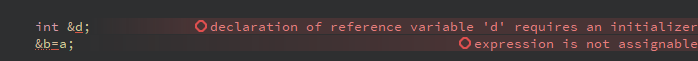2. 指针

指针是一个变量，其值为另一个变量的地址。

1. 定义一个指针变量
2. 把变量地址赋值给指针
3. 访问指针变量中可用地址的值。

3. 引用

给一个已经定义好的变量去个别名。

int main() {
int a = 10;
int &b = a;
int &c = b;
std::cout << "a:  " << &a << "  " << a << std::endl;
std::cout << "b:  " << &b << "  " << b << std::endl;
c = 100;
std::cout << "a:  " << &a << "  " << a << std::endl;
std::cout << "b:  " << &b << "  " << b << std::endl;
std::cout << "c:  " << &c << "  " << c << std::endl;
}

a:  0000002106D8FD80  10
b:  0000002106D8FD80  10
a:  0000002106D8FD80  100
b:  0000002106D8FD80  100
c:  0000002106D8FD80  100

4. 引用&&指针 作为参数

指针和引用作为参数传入函数一样，都是：隐式的返回传递值

void Quote(int &x) {
std::cout << "x:  " << &x << "  " << x << std::endl;
x++;
std::cout << "x:  " << &x << "  " << x << std::endl;
}

void Pointer(int *x) {
std::cout << "x:  " << &x << "  " << x << "  " << *x << std::endl;
x++;
std::cout << "x:  " << &x << "  " << x << "  " << *x << std::endl;
x++;
std::cout << "x:  " << &x << "  " << x << "  " << *x << std::endl;
}

void Normal(int x) {
std::cout << "x:  " << &x << "  " << x << std::endl;
x++;
std::cout << "x:  " << &x << "  " << x << std::endl;
}

int main() {
int a = 10;
std::cout << "a:  " << &a << "  " << a << std::endl;
std::cout << "Quote-----------" << std::endl;
Quote(a);
std::cout << "a:  " << &a << "  " << a << std::endl;
std::cout << "Pointer-----------" << std::endl;
Pointer(&a);
std::cout << "a:  " << &a << "  " << a << std::endl;
std::cout << "Normal-----------" << std::endl;
Normal(a);
std::cout << "a:  " << &a << "  " << a << std::endl;
}

a:  000000B7B8CFF8B0  10
Quote-----------
x:  000000B7B8CFF8B0  10
x:  000000B7B8CFF8B0  11
a:  000000B7B8CFF8B0  11
Pointer-----------
x:  000000B7B8CFF8B8  000000B7B8CFF8B0  11
x:  000000B7B8CFF8B8  000000B7B8CFF8B0  12
x:  000000B7B8CFF8B8  000000B7B8CFF8B4  0
a:  000000B7B8CFF8B0  12
Normal-----------
x:  000000B7B8CFF8B8  12
x:  000000B7B8CFF8B8  13
a:  000000B7B8CFF8B0  12

结论：建议使用引用，看着舒服，而且效率高一些。

翻译成汇编后，指针传递比参数多一个创建变量。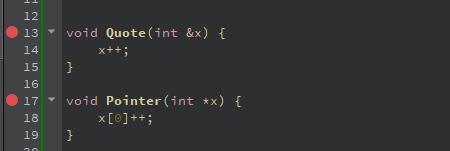1_17!Quote [f:\studyc\1-17\main.cpp @ 13]:
0x7ff7534f2a60                   mov     qword ptr [rsp+8],rcx
0x7ff7534f2a65  <+    5>         push    rdi
0x7ff7534f2a66  <+    6>         mov     rax,qword ptr [rsp+10h]
0x7ff7534f2a6b  <+   11>         mov     eax,dword ptr [rax]
0x7ff7534f2a6d  <+   13>         inc     eax
0x7ff7534f2a6f  <+   15>         mov     rcx,qword ptr [rsp+10h]
0x7ff7534f2a74  <+   20>         mov     dword ptr [rcx],eax
0x7ff7534f2a76  <+   22>         pop     rdi
0x7ff7534f2a77  <+   23>         ret

1_17!Pointer [f:\studyc\1-17\main.cpp @ 17]:
0x7ff7534f2a80  <+   32>         mov     qword ptr [rsp+8],rcx
0x7ff7534f2a85  <+   37>         push    rdi
0x7ff7534f2a86  <+   38>         mov     eax,4
0x7ff7534f2a8b  <+   43>         imul    rax,rax,0
0x7ff7534f2a8f  <+   47>         mov     rcx,qword ptr [rsp+10h]
0x7ff7534f2a94  <+   52>         mov     eax,dword ptr [rcx+rax]
0x7ff7534f2a97  <+   55>         inc     eax
0x7ff7534f2a99  <+   57>         mov     ecx,4
0x7ff7534f2a9e  <+   62>         imul    rcx,rcx,0
0x7ff7534f2aa2  <+   66>         mov     rdx,qword ptr [rsp+10h]
0x7ff7534f2aa7  <+   71>         mov     dword ptr [rdx+rcx],eax
0x7ff7534f2aaa  <+   74>         pop     rdi

4.1. 常引用

int a;
const int &ra=a;
ra=1; //错误
a=1; //正确

常引用无法修改变量本身的值。使用引用作为参数传入效率高

引用型参数应该在能被定义为const的情况下，尽量定义为const。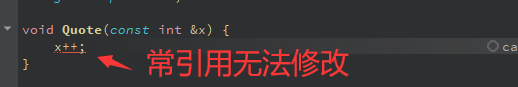5. 把引用&&指针 作为返回值

通过使用引用来替代指针，会使 C++ 程序更容易阅读和维护。返回指针的话可以表示指针的数组名。

int value[] = {0, 1, 2, 3};

int *GetPointerValue(int i) {
return &value[i];
}

int *GetPointersValue() {
int *r = new int;
for (int i = 0; i < 4; i++) {
r[i] = value[i];
}
return r;
}

int &GetQuoteValue(int i) {
return value[i];
}

int main() {
std::cout << value << std::endl;
*GetPointerValue(0) = -1;
std::cout << value << std::endl;
GetQuoteValue(0) = -2;
std::cout << value << std::endl;
int *r = GetPointersValue();
std::cout << r << " " << r << " " << r << std::endl;
}

0
-1
-2
-2 1 2

引用返回局部变量不合法的，下边能编译通过，而且测试运行正常，但是如果b所在内存给其他再次使用过，那程序就炸了。6. 引用&&指针 实现多态

这俩也没啥大区别。

class A {
};
class B: public A {
};

int main() {
B a;
A *aa = new B;
A &aaa = a;
}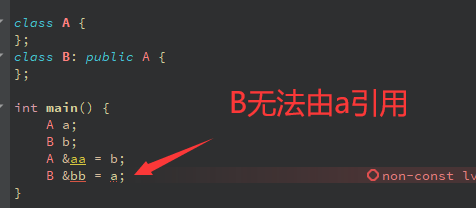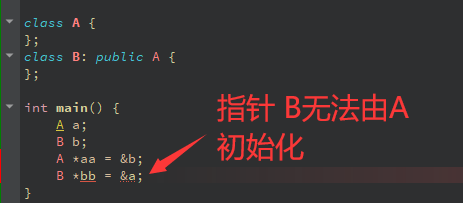展开全文• C++指针的使用

2021-10-15 20:01:31
一、指针的定义和使用 可以通过指针来保存一个变量的地址 例如： int a=2; 就相当于内存中分出了一个内存块给变量a，而这个内存块中储存的数值为2； 假设这个内存块的地址为0x2e； 则可以通过定义一个指针来储存这...c++ c语言 数据结构
• 指针占用空间 32位操作系统下指针占用四个字节的空间. 64位下占用8个字节. const 指针组合 const修饰指针 --- 常量指针 声明 const T *p = xxx; 特点 指针指向可以修改, 但是指针指向的值不可以修改. 比如: ...c++ 指针
• C++指针(三): 指针与二维数组 在介绍指针与二维数组之前，先介绍二维数组的地址、行地址以及元素地址。c++ 指针 数据结构
• 首先,当指针作为形参时,实参向形参传递的是地址,在函数执行过程中既可以对指针变量进行操作也可以对指针所指数据进行操作(即P和*P).由于形参和实参指向同一个内存单元,当形参指向的数据改变那么实参所指向的数据也...
• C++中我们可以通过指针方便的访问各种类型数据，但我们都知道指针只是一个头地址，而我们需要遍历的数据通常占有大量的空间，那么指针是如何遍历其中的数据呢？这就涉及到指针偏移的一个问题，下面我们看个例子： ...c++
• c++中可以将引用和指针来作为返回的参数，但是当将指针作为返回参数的时候，犯了一个错误。导致程序fault。 错误的示例： void getData(unordered_map<int, int> sourceData, int* target2) { if (sourceData...c++
• 1. 指针的定义和使用方式 int a = 10; int *p = &a; *p = 100; 2. 指针所占的内存空间 32位系统为四个字节（无论指向的数据类型是什么）， 64为系统为8个字节。 3. 空指针 int* p = NULL; int* p = ...c++
• int* func()//返回值是地址 { int* p = new int(10);...指针变量p1拿到func函数的返回地址，指针变量p销毁； cout << *p1 << endl;刚刚在堆区new的数据的地址被p1拿到； int a=10; int *p2=a; rec++ 指针
• C++指针常量和常量指针 指针常量 #include <iostream> using namespace std; int main() { int a = 1; int b = 2; int * const c = &a;//c指向a，c是指针常量，c不可被修改 c = &b;//错误，c被...
• C++指针(二): 指针与数组 在C++中，指针与数组具有非常多的联系，在学习指针与数组的操作之前，我们先简单复习一下C++中的数组。 一维数组 #include <iostream> int main() { int a = {1,2,3,4,5}; std...c++ 算法 指针
• C++指针和引用因为涉及到地址和地址当中的存储内容，所以比较容易混淆。首先需要注意的是，指针和引用存放的都是被引用对象的地址！地址！地址！比如对于指针，当我们定义一个指针p，比如说int* p = &a时，...c++
• c++指针后移

2021-06-25 10:53:08
int a=10; int *p=&a; cout<<++p<<endl;...若a的地址为100，p++之后，p不是指向101，而是104（32位系统），108（64位系统）。...对指针后移++，默认是对类型++后移，指针加上类型的长度
• #include<iostream> #include<string> using namespace std; //定义结构体 struct Student { string name;... //结构体指针 ... //通过指针c语言访问... //创建和结构体的类型相同的指针 Student* p = &.
• C++中数组名和指针基本等价 大家好，在下康宁。 本文深度解释了C++中数组和指针的关系。 话不多说，先上代码： #include<iostream> int main() { using namespace std; double wages = {1.0,2.0,3.0}; ...指针 c++
• 链表 链表是一种线性的数据结构，但是其内存空间和数组不同，是不连续的，因此...位置信息采用指针来实现。具体设计的代码如下： template<typename T> class Node{ public: T value; Node<T> *next;数据结构 链表 c++ 算法
• CSAPP第三章看到3.8了，这一节在讲关于数据的表示，其中提到了指针的运算，本来自己只是想实践一下加深点印象，没想到小小的测试程序还又学到了一些新知识，觉得有必要记录一下： 1、首先是正题，关于指针的加减法，...
• 指针在程序中起到传递变量地址、优化内存调用的作用，为变量/对象声明指针后，指针承载了变量/对象的内存地址。c++ c语言
• 在这里我们将会为大家详细介绍一下有关C++指针漂移的解决方法，希望本文介绍的内容可以帮助大家解决问题。最近我们在工作中碰到一个奇怪的问题，最后确定是多继承引起的C++指针漂移，跟C++对象模型有关。示意如下：...
• 1.一级指针和二级指针 一级指针即常说的指针，其保存内存地址； 二级指针即指向指针指针，就是保存内存地址的指针变量的地址； 例： int a=1; int *p=&a;　// p为a变量的地址，通过*p可以得到a的值 int **q=&...c++ 指针
• 举个栗子：删除列表中的某个节点： 表示法1： /** * Definition for singly-linked list. * struct ListNode { * int val; * ListNode *next;... * ListNode(int x) : val(x), next(NULL) {} ...经验分享 c++
• 1.由于引用不是变量，所以不能声明引用的引用，也不能声明数组元素的类型为引用或者指向引用的指针。例如： int &(&a);//错误 int &&a = 10;//正确，这是右值引用，可以修改 int &a;//错误 ...c++ 指针
• c++中的引用是给变量取别名 类型名& 别名=变量名； int& newname =a; 引用和指针的区别 1 引用是某块地址的别名，不分配新的空间 指针是存储所指内存的地址，大小四个字节 2 “sizeof 引用”得到的是所指向...指针 引用传递
• 指针 函数的参数不仅可以是整数、小数、字符等具体的数据，还可以是指向它们的指针。 用指针变量作函数参数可以将函数外部的地址传递到函数内部，使得在函数内部可以操作函数外部的数据，并且这些数据不会随着函数的...
• int i=5; int &r=i; //r是一个引用 int *p; //p是一个指针 p=&i ; //&是一个取地址符 *p=i ; // * 是一个解引用符 int &r2= *p // 都是对象i ，i的值发生变化，r、r2、*p 一起变。
• 取地址 int *b=&a 和变量在一起 引用 int &c=a 和类型在一起，相当于a有个别名叫c，以后都用c计算实际改变的是a所在内存的值 int &b=a； //引用 ...注意结构体指针访问变量时加括号 \ ⭐(sc++ c语言
• 在C语言中，不同类型占用多少字节非常重要，很多指针的操作就是在理解不同类型的字节数大小后进行操作的 在实际计算机处理数据中，地址和变量是分不开，而指针变量就是记录地址的变量 访问方式 直接访问就是指用...c++ c语言 c#...

c++指针c++ 订阅